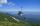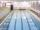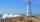Average climb of the road is given by ratio 1:15. By what angle road average climb?

Result

α =  3.81 °

#### Solution:Leave us a comment of example and its solution (i.e. if it is still somewhat unclear...):Be the first to comment!## Next similar examples:

1. ClimbRoad has climbing 1:27. How big is a angle corresponds to this climbing?On a straight stretch of road is marked 12 percent drop. What angle makes the direction of the road with the horizontal plane?
3. Map - climbOn the map of High Tatras in scale 1:11000 are cable car stations in the Tatranska Lomnica and in the Skalnate Pleso with distance 354.6 mm. Altitude of this stations are 949 m and 1760 m. What is average angle of climb of this cable car track?
4. StairwayWhat angle rising stairway if step height in 17 cm and width 27 cm?
5. BevelI have bevel in the ratio 1:6. What is the angle and how do I calculate it?
6. AnglesIn the triangle ABC, the ratio of angles is: a:b = 4: 5. The angle c is 36°. How big are the angles a, b?
7. MedianThe median of the triangle LMN is away from vertex N 84 cm. Calculate the length of the median, which start at N.
8. ReflectorCircular reflector throws light cone with a vertex angle 49° and is on 33 m height tower. The axis of the light beam has with the axis of the tower angle 30°. What is the maximum length of the illuminated horizontal plane?
9. FlowerbedFlowerbed has the shape of an isosceles obtuse triangle. Arm has a size 5.5 meters and an angle opposite to the base size is 94°. What is the distance from the base to opposite vertex?
10. Theorem proveWe want to prove the sentence: If the natural number n is divisible by six, then n is divisible by three. From what assumption we started?
11. Slope of the poolCalculate slope (rise:run) of the bottom of swimming pool long 10 m. Water depth at beginning of pool is 1.16 m (for children) and depth at end is 1.89 m (for swimmers). Slope express as percentage and as angle in degrees.
12. How farFrom the top of a lighthouse 145 ft above sea level, the angle of depression of a boat 29°. How far is the boat from the lighthouse?
13. TreeHow tall is the tree that observed in the visual angle of 52°? If I stand 5 m from the tree and eyes are two meters above the ground.
14. High wallI have a wall 2m high. I need a 15 degree angle (upward) to second wall 4 meters away. How high must the second wall?
15. MapleMaple peak is visible from a distance 3 m from the trunk from a height of 1.8 m at angle 62°. Determine the height of the maple.
16. Reference angleFind the reference angle of each angle:
17. Center traverseIt is true that the middle traverse bisects the triangle?